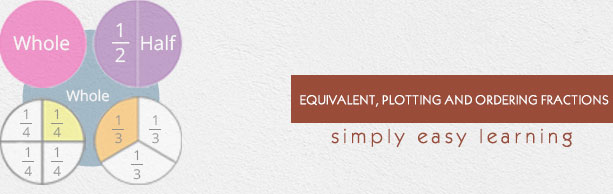# Equivalent Plotting And Ordering Fractions

This tutorial provides comprehensive coverage of equivalent, plotting and ordering fractions based on Common Core (CCSS) and State Standards and its prerequisites. Students can navigate learning paths based on their level of readiness. Institutional users may customize the scope and sequence to meet curricular needs. This simple tutorial uses appropriate examples to help you understand equivalent, plotting and ordering fractions in a general and quick way.

# Audience

This tutorial has been prepared for beginners to help them understand the basics of equivalent, plotting and ordering fractions. After completing this tutorial, you will find yourself at a moderate level of expertise in equivalent, plotting and ordering fractions, from where you can advance further.

# Prerequisites

Before proceeding with this tutorial, you need a basic knowledge of elementary math concepts such number sense, multiplication, division, whole numbers, place values and ordering of whole numbers, fractions, number line and so on.